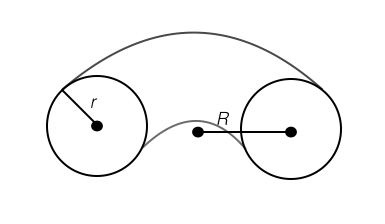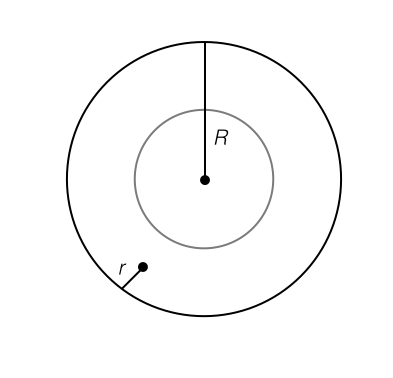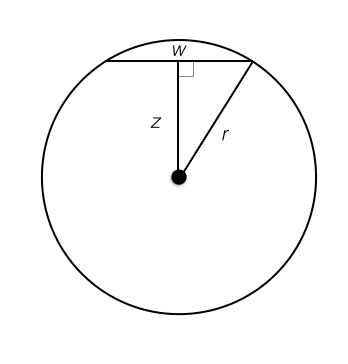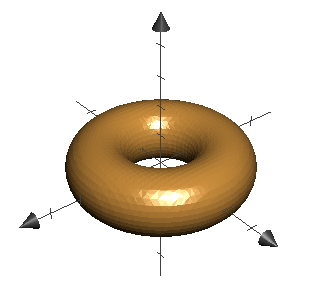## How to Graph a Donut

2017 Jan 28

What is the equation of the graph of a torus in the 3D coordinate plane?

Suppose one wants to graph a torus with major radius $R$ (the distance from the middle of the torus from the center of one of the circles forming its body) and minor radius $r$ (the radius of one of the small circles forming the body of the torus) in the three-dimensional coordinate plane. If one vertically sliced it in half down the middle, it would look something like this:If one sliced it horizontally down the middle, it would look like this:Each horizontal plane cross section consists of two circles (except for the one made by the tangent planes at the top and bottom), one inside of the other. Let the difference between their radii at height $z$ be the width $w$. The graph of a circle has the equation Where $a$ is the radius of the circle. However, we want two circles with a middle circle of radius $R$ and a radius difference $w$, so the new equation is or Now I need to express $w$ as a function of $z$, the height. Again, we observe a vertical cross-section of the torus:From the picture, we can see that by the pythagorean theorem. By solving for $w$, we obtain Now we can plug this in for $w$ in our formula for a horizontal donut cross-section: And this is our formula for the graph of a torus. Essentially what we did was figure out what one "layer" of the donut looks like at some height $z$ and then stack up infinitely many layers for each value of $z$ to make a smooth donut. Here is our hard-earned donut:Yum!